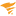cancel
Showing results for
Did you mean:
Level 10

## Log Parser (Powershell) accept regex expressions

Is there a way to allow the Log Parser (Powershell) to accept more complex regex expressions such as the following: ^.*(\\sERROR\\s).*\$

Any help would be appreciated, I feel like I'm spinning my wheels here. Thanks!

Tags (1)Product Manager
 jdwinns  wrote:Is there a way to allow the Log Parser (Powershell) to accept more complex regex expressions such as the following: ^.*(\\sERROR\\s).*\$Any help would be appreciated, I feel like I'm spinning my wheels here. Thanks!

Hi JD,

I applied the Log Parser (Powershell) template to my lab and just grabbed the powershell script to test your regex by replacing the first two arguments. It works fine on the the example with Total number of strings found:

# Arguments example: d:\powertest.txt,string

\$logfile_path = "C:\Windows\tracing\logfile.log.txt";

\$regex = "^.*(\\sERROR\\s).*\$";

\$Error.Clear();

if ( \$logfile_path -eq \$null )

{

Write-Host "Message: Can't find ""logfile_path"" argument. Check documentation."

exit 1

}

if ( \$regex -eq \$null )

{

Write-Host "Message: Can't find ""regex"" argument. Check documentation."

exit 1

}

if ( !\$(Test-Path \$logfile_path) )

{

exit 1

}

\$filename = split-path "\$logfile_path" -leaf

\$t = (Get-Childitem env:temp).value

\$usage="total";

\$regname = [System.Text.RegularExpressions.Regex]::Replace(\$regex,"[^1-9a-zA-Z_]","_");

\$txt=".txt"

\$file_path = "\$t\\$usage-\$filename-\$regname-\$txt"

if ( Test-Path \$file_path )

{ \$known_rows = get-content \$file_path }

else

{ \$known_rows = 0 }

\$resn = @()

\$resl = @()

\$matching_rows = get-childitem \$logfile_path | select-string -pattern \$regex

\$total = \$matching_rows.Count

if (\$Error.Count -ne 0) {

Write-Host "\$(\$Error)"

exit 1

}

if ( \$total -lt \$known_rows ) {

\$known_rows = 0

\$known_rows > \$file_path

}

\$new_rows = \$total - \$known_rows

\$total > \$file_path

for ( \$i = 0 ; \$i -le \$total; \$i++ ) {

\$resn += @(\$i)

\$resl += @(\$i) }

\$i = 1

\$stat = \$matching_rows | select linenumber, line | ForEach-Object {

\$resn[\$i] = \$_.linenumber

\$resl[\$i] = \$_.line

\$i = \$i + 1

}

if (\$total -eq 0)

{

write-host "Statistic: 0"

write-host "Message: No strings found"

exit 0

}

if (\$total -gt 0)

{

\$lines = @()

write-host "Statistic: \$total"

if(\$total -gt 1)

{

for ( \$i = 1 ; \$i -le \$total; \$i++ )

{

\$lines += "<br/>"

\$lines += \$resl[\$i]

\$lines += ";"

}

write-host "Message: Total number of lines that match search criteria: \$total. Lines that have search string: \$lines"

exit 0

}

else

{

\$line = \$resl[\$resl.Count - 1]

write-host "Message: Total number of lines that match search criteria: \$total. Lines that have search string: \$line"

exit 0

}

}

Running this script in powershell returned the correct number: 0 for me. As this is the script that's used by the Log Parser template, there should be no limitations on using your regex that you listed. Have you tried again? what error are you seeing? Is it in all of the components in this template?